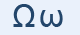Greek Letter Omega (Ωμέγα - Ω)"Big o". It was marked specially by a bar under the upper case, doubling the lower case. This letter was not introduced in the Latin alphabet, but survives as the symbol for electric resistance (ohms). The apocalypse has the Lord say, "I am Alpha and Omega, the beginning and the end." Tielhard de Chardin, the priest-archeologist and mystic who discovered Peking Man, saw evolution reaching the "omega point".

"Ω" Letter in mathematics and in science

Ω represents:

• the Omega constant
• an asymptotic lower bound related to Big O notation
• in probability theory and statistical mechanics, the set of possible distinct system states
• the SI unit measure of electric resistance, the ohm
• the rotation rate of an object, particularly a planet, in dynamics
• a solid angle
• a baryon

ω represents:

• the first infinite ordinal
• an asymptotically dominant quantity related to Big O notation
• in probability theory, a possible outcome of an experiment
• angular velocity / radian frequency
• a complex cube root of unity - the other is ω2
• vertical velocity in pressure-based coordinate systems (commonly used in atmospheric dynamics)
• a meson

Greek words with "ω"

ωραίο, ψωμί